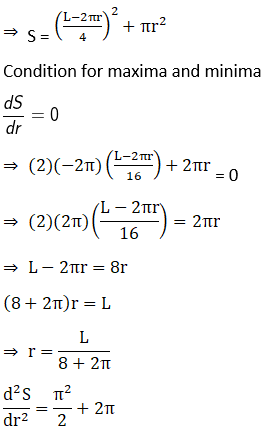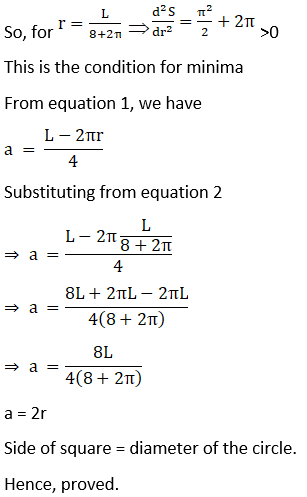Given the sum of the perimeters of a square and a circle, show that the sum of their areas is least when one side of the square is equal to diameter of the circle.

Asked by Aaryan | 1 year ago |  135

##### Solution :-

Let us say the sum of perimeter of square and circumference of circle be L

Given sum of the perimeters of a square and a circle.

Assuming, side of square = a and radius of circle = r

Then, L = 4a + 2πr

= a = $$\dfrac{ (L – 2πr)}{4}$$… (1)

Let the sum of area of square and circle be S

So, S = a2 + πr2Answered by Sakshi | 1 year ago

### Related Questions

#### A wire of length 20 m is to be cut into two pieces. One of the pieces will be bent into shape

A wire of length 20 m is to be cut into two pieces. One of the pieces will be bent into shape of a square and the other into shape of an equilateral triangle. Where the wire should be cut so that the sum of the areas of the square and triangle is minimum?

#### A wire of length 28 m is to be cut into two pieces. One of the pieces is to be made into a square

A wire of length 28 m is to be cut into two pieces. One of the pieces is to be made into a square and the other into a circle. What should be the lengths of the two pieces so that the combined area of the circle and the square is minimum?

#### Of all the closed cylindrical cans (right circular), which enclose a given volume of 100 cm3, which has the minimum

Of all the closed cylindrical cans (right circular), which enclose a given volume of 100 cm3, which has the minimum surface area?

How should we choose two numbers, each greater than or equal to –2, whose sum is $$\dfrac{1}{2}$$ so that the sum of the first and the cube of the second is minimum?